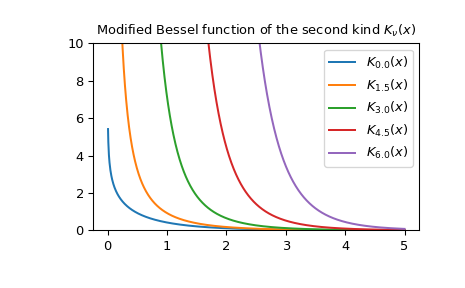# scipy.special.kv¶

scipy.special.kv(v, z) = <ufunc 'kv'>

Modified Bessel function of the second kind of real order v

Returns the modified Bessel function of the second kind for real order v at complex z.

These are also sometimes called functions of the third kind, Basset functions, or Macdonald functions. They are defined as those solutions of the modified Bessel equation for which,

$K_v(x) \sim \sqrt{\pi/(2x)} \exp(-x)$

as $$x \to \infty$$ [R527].

Parameters: v : array_like of float Order of Bessel functions z : array_like of complex Argument at which to evaluate the Bessel functions out : ndarray The results. Note that input must be of complex type to get complex output, e.g. kv(3, -2+0j) instead of kv(3, -2).

kve
This function with leading exponential behavior stripped off.
kvp
Derivative of this function

Notes

Wrapper for AMOS [R525] routine zbesk. For a discussion of the algorithm used, see [R526] and the references therein.

References

 [R525] (1, 2) Donald E. Amos, “AMOS, A Portable Package for Bessel Functions of a Complex Argument and Nonnegative Order”, http://netlib.org/amos/
 [R526] (1, 2) Donald E. Amos, “Algorithm 644: A portable package for Bessel functions of a complex argument and nonnegative order”, ACM TOMS Vol. 12 Issue 3, Sept. 1986, p. 265
 [R527] (1, 2) NIST Digital Library of Mathematical Functions, Eq. 10.25.E3. http://dlmf.nist.gov/10.25.E3

Examples

Plot the function of several orders for real input:

>>> from scipy.special import kv
>>> import matplotlib.pyplot as plt
>>> x = np.linspace(0, 5, 1000)
>>> for N in np.linspace(0, 6, 5):
...     plt.plot(x, kv(N, x), label='$K_{{{}}}(x)$'.format(N))
>>> plt.ylim(0, 10)
>>> plt.legend()
>>> plt.title(r'Modified Bessel function of the second kind $K_\nu(x)$')
>>> plt.show()Calculate for a single value at multiple orders:

>>> kv([4, 4.5, 5], 1+2j)
array([ 0.1992+2.3892j,  2.3493+3.6j   ,  7.2827+3.8104j])


scipy.special.kn

#### Next topic

scipy.special.kve